### Piecewise Interpolation: Piecewise Linear Spline Interpolation

### Piecewise Linear (Piecewise Affine) Spline Interpolation

Given a set of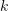data points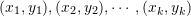, a piecewise linear (piecewise affine) spline can be defined as: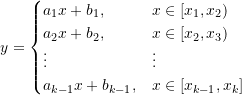Thedata points have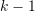intervals. The linear function for each interval is defined using two coefficients, and therefore, we need to find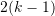coefficients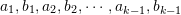. The coefficients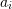and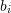can be found by solving the two equations: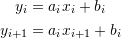Therefore: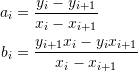#### Example

Consider the following 11 data points: (-1.00,0.038),(-0.80,0.058),(-0.60,0.100),(-0.4,0.200),(-0.20,0.500),(0.00,1.000),(0.20,0.500),(0.40,0.200),(0.60,0.100),(0.80,0.0580),(1.00,0.038) which were generated using the Runge function. Find the explicit representation of the linear spline interpolating function for these data points.

##### Solution

There are 11 data points surrounding 10 intervals. For each interval, a set of coefficientsandare required for the linear interpolation. For the first interval, the coefficients are: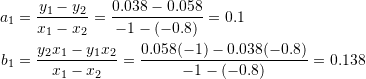Similarly: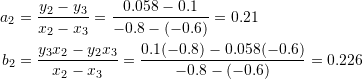Repeating for the other coefficients, the required explicit equation has the form: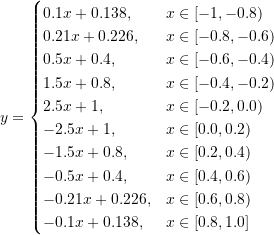Notice that for the procedure to work, thecomponents of the data points have to satisfy: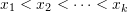. The following Mathematica code utilizes a user defined procedure “Spline1” that creates the required piecewise linear function.

View Mathematica Code
Data = {{-1, 0.038}, {-0.8, 0.058}, {-0.60, 0.10}, {-0.4,0.20}, {-0.2, 0.50}, {0, 1}, {0.2, 0.5}, {0.4, 0.2}, {0.6,0.1}, {0.8, 0.058}, {1, 0.038}};
Spline1[Data_] := (
k1 = Length[Data] - 1;
atable = Table[(Data[[i, 2]] - Data[[i + 1, 2]])/(Data[[i, 1]] -Data[[i + 1, 1]]), {i, 1, k1}];
btable = Table[(Data[[i + 1, 2]]*Data[[i, 1]] -Data[[i, 2]]*Data[[i + 1, 1]])/(Data[[i, 1]] - Data[[i + 1, 1]]), {i, 1, k1}];
pf = Table[{atable[[i]] x + btable[[i]],Data[[i, 1]] <= x <= Data[[i + 1, 1]]}, {i, 1, k1}];
Piecewise[pf]
)
y = Spline1[Data]
a = Plot[{y, yactual}, {x, -1, 1}, Epilog -> {PointSize[Large], Point[Data]}, AxesLabel -> {"x", "y1"}, ImageSize -> Medium, PlotRange -> All,  PlotLegends -> {"y1", "yactual"}, PlotLabel -> "Linear splines"]

View Python Code
import numpy as np
import matplotlib.pyplot as plt
Data = [[-1, 0.038], [-0.8, 0.058], [-0.60, 0.10], [-0.4,0.20], [-0.2, 0.50], [0, 1], [0.2, 0.5], [0.4, 0.2], [0.6, 0.1], [0.8, 0.058], [1, 0.038]]
def Spline1(x, Data):
k1 = len(Data) - 1
atable = [(Data[i] - Data[i + 1])/(Data[i] - Data[i + 1]) for i in range(k1)]
btable = [(Data[i + 1]*Data[i] - Data[i]*Data[i + 1])/(Data[i] - Data[i + 1]) for i in range(k1)]
display([['{:15}'.format(str(round(atable[i],3))+'x + '+str(round(btable[i],3))),
'{:15}'.format(str(round(Data[i],3))+' <=x<= '+str(round(Data[i + 1],3)))] for i in range(k1)])
return np.piecewise(x, [(x >= Data[i])&(x <= Data[i + 1]) for i in range(k1)],
[lambda x, j=i: atable[j]*x + btable[j] for i in range(k1)])
x = np.arange(-1,1,0.01)
y = Spline1(x, Data)
yactual = 1/(1 + 25*x**2)
plt.plot(x,yactual, label="yactual")
plt.plot(x, y, label="y1")
plt.scatter([point for point in Data],[point for point in Data], c='k')
plt.title("Linear splines")
plt.xlabel("x"); plt.ylabel("y1")
plt.legend(); plt.grid(); plt.show()